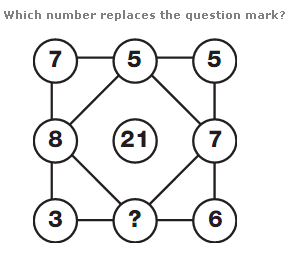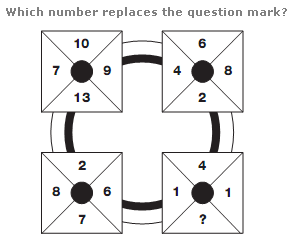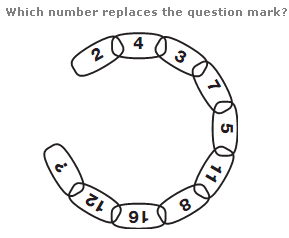# Puzzles - Number puzzles

### Exercise :: Number puzzlesAnswer : 1 Explanation : The four numbers in the corners of the square, and the four numbers in the middle of each side of the square add up to 21.Answer : 4 Explanation : Add up numbers in corresponding segments of each square. The sum of the digits in the left hand segments equals 20, the sum of the upper segments equals 22, the right hand segments equals 24 and the lower segments equal 26.Answer : 22 Explanation : There are two repeated sequences in the circle. Starting with the first segment and moving clockwise, add 1 to the first number, then 2, 3, and 4, writing the results in alternate segments. Starting with the second segment, add 3 to this number, then 4, 5 and 6, writing these results in the alternate segments left.